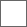### 【原创】诗词游戏联想对对碰，关键字为女明星的名字（第二场）（）（）（）（）（）金（），（）（）
（）（）（）（）（）。

（）（）（）孙（），（）（）（）（）（）。

（）（）（）怡（），（）（）（）（）（）。

（）（）（）（）（）（）（），（）（）（）（）（）（）乔。

（）（）（）欣（），（）（）（）（）（）。

（）（）（）（）（）（）？杨（）（），（）（）（）（）。

（）（）（）（）（）（）（），（）紫（）（）（）（）（）。

（）赵（）（）（），（）（）（）（）（）。

#### 扫二维码下载贴吧客户端